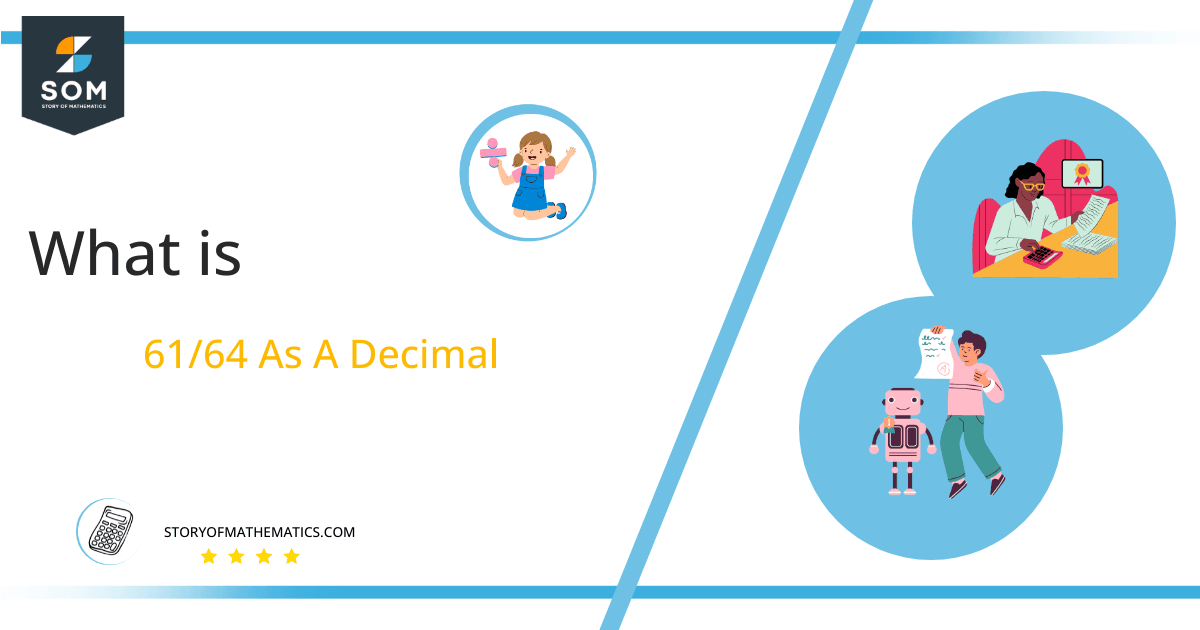# What Is 61/64 as a Decimal + Solution With Free Steps

The fraction 61/64 as a decimal is equal to 0.953.

Fractional results of a division operation are the most common and simplified results of division that are used in mathematics. Furthermore, these fractional values, are easy to understand and use in other mathematical equations for further calculations.

Here, we are more interested in the division types that result in a Decimal value, as this can be expressed as a Fraction. We see fractions as a way of showing two numbers having the operation of Division between them that result in a value that lies between two Integers.Now, we introduce the method used to solve said fraction to decimal conversion, called Long Division, which we will discuss in detail moving forward. So, let’s go through the Solution of fraction 61/64.

## Solution

First, we convert the fraction components, i.e., the numerator and the denominator, and transform them into the division constituents, i.e., the Dividend and the Divisor, respectively.

This can be done as follows:

Dividend = 61

Divisor = 64

Now, we introduce the most important quantity in our division process: the Quotient. The value represents the Solution to our division and can be expressed as having the following relationship with the Division constituents:

Quotient = Dividend $\div$ Divisor = 61 $\div$ 64

This is when we go through the Long Division solution to our problem. Given is the long division process in Figure 1:Figure 1

## 61/64 Long Division Method

We start solving a problem using the Long Division Method by first taking apart the division’s components and comparing them. As we have 61 and 64, we can see how 61 is Smaller than 64, and to solve this division, we require that 61 be Bigger than 64.

This is done by multiplying the dividend by 10 and checking whether it is bigger than the divisor or not. If so, we calculate the Multiple of the divisor closest to the dividend and subtract it from the Dividend. This produces the Remainder, which we then use as the dividend later.

Now, we begin solving for our dividend 61, which after getting multiplied by 10 becomes 610.

We take this 610 and divide it by 64; this can be done as follows:

610 $\div$ 64 $\approx$ 9

Where:

64 x 9 = 576

This will lead to the generation of a Remainder equal to 610 – 576 = 34. Now this means we have to repeat the process by Converting the 34 into 340 and solving for that:

340 $\div$ 64 $\approx$ 5

Where:

64 x 5 = 320

This, therefore, produces another Remainder which is equal to 340 – 320 = 20. Now we must solve this problem to Third Decimal Place for accuracy, so we repeat the process with dividend 200.

200 $\div$ 64 $\approx$ 3

Where:

64 x 3 = 192

Finally, we have a Quotient generated after combining the three pieces of it as 0.953, with a Remainder equal to 8.Images/mathematical drawings are created with GeoGebra.